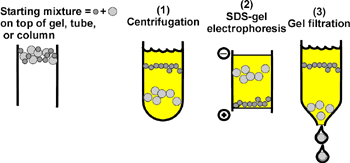Methods based on size for separation of large molecules

1. Centrifugation

When you put a particle in a centrifugal field, it is acted upon by the centrifugal force, which is proportional to the molecular weight (M), to the square of the speed (angular velocity, rpm) of the rotor (w2) and to the distance of the solution from the center of rotation (r): Cent. Force ~ Mw2r. Acting in the opposite direction to particle motion is friction. This frictional force is proportional to the velocity of the particle (V) and to a coefficient of friction (f) that depends on the shape of the particle: Friction force ~ fV. The particle will accelerate until a velocity is achieved such that these two opposing forces are equal, after which the particle will continue to sediment, but at a constant velocity. Setting the 2 forces equal to each other we have: Mw2r=fV, and so:

V ~ Mw2r/f.

(NOTE that this equation was printed incorrectly with w2r in the denominator on the hard copy of this handout).

In general, this means that velocity increases as mass (molecular weight) increase, and velocity decreases as the cross-sectional size (diameter) increases (f increases with cross-sectional size).

V depends on the particular machine and on the speed of rotation. So you can compare results irregardless of machine and speed, you calculate the Svedberg constant or S value instead of V. S = V/w2r. Macromolecules usually have S values betw een 1 and 100 X 10-13 sec. A value of 1 X 10-13 sec is called one Svedberg unit or S. Many molecules are known by their S values, for example 23 S ribosomal RNA. Its S value was known before its function or molecular weight, and so has become its name.

Molecules of different S values are usually separated by layering them on top of a dense solution (such as sucrose) and then running the centrifuge to force the molecules through the solution. The molecules of larger S value go farther because of eithe r larger molecular weight or less frictional drag or both. You stop the centrifuge when the different molecules have traveled different distances down the tube and then remove the solution by puncturing the bottom of the tube or pumping the solution off t he top. The invention of the ultracentrifuge (a super fast centrifuge) by Svedberg was what originally made the purification of macromolecules possible. Today there are many other methods also used, as listed below.

2. Gel Electrophoresis plus SDS

When proteins and other macromolecules are treated with SDS, a strong detergent, they are denatured. They also become negatively charged because of the charge on the detergent, and the amount of detergent bound is so large that any differences in n ative charge are swamped. The bigger the macromolecule, the more SDS is bound, so that all macromolecules treated with SDS have the same ratio of charge to mass. (The amount bound is proportional to mass.) So for molecules treated with SDS, the pull per u nit mass in an electric field is the same, and all molecules should have the same velocity IF there is no frictional drag. But electrophoresis with SDS is always done in a gel, so that the molecules must be pulled though the pores. And the ease of moving through the pores depends on the diameter of the molecules. The bigger molecules are retarded or keep getting stuck and don't move as fast. Since the molecules are all denatured into random coils, the diameter strictly depends on the length or molecular w eight. The bigger the molecular weight, the longer the coil and the slower the molecule goes. So, electrophoresis + SDS separates on the basis of molecular weight, not on the basis of native charge. Important note: Proteins of the same length usually cann ot be separated by gel electrophoresis + SDS. The differences in molecular weight caused by differences in the R groups are not enough to allow a separation. If two proteins migrate the same +SDS they are assumed to be of approximately the same molecular weight because they are about the same length (contain the same # of amino acids).

3. Gel filtration or molecular sieve chromatography